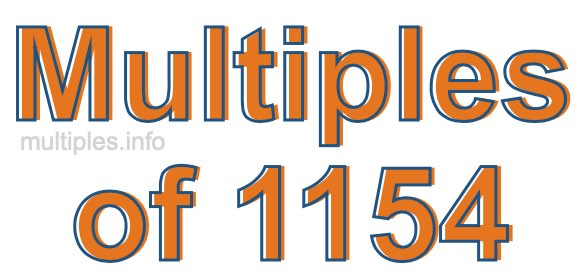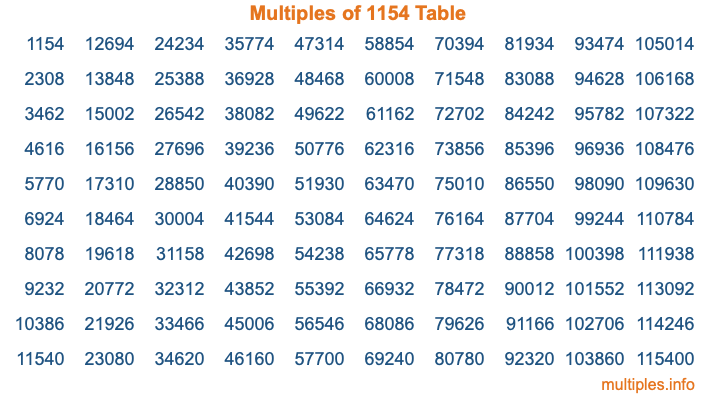Multiples of 1154Welcome to the Multiples of 1154 page. Here we will first teach you everything you will ever need to know about the multiples of 1154, and then give you a study guide summary of everything we taught you to make sure you remember it all. Use this page to look up facts and learn information about the multiples of 1154. This page will make you a multiples of one thousand one hundred fifty-four expert!

Definition of Multiples of 1154
Multiples of 1154 are all the numbers that when divided by 1154 equal an integer. Each of the multiples of 1154 are called a multiple. A multiple of 1154 is created by multiplying 1154 by an integer.

Therefore, to create a list of multiples of 1154, you start with 1 multiplied by 1154, then 2 multiplied by 1154, then 3 multiplied by 1154, and so on for as long as you want. Thus, the list of the first five multiples of 1154 is 1154, 2308, 3462, 4616, and 5770. To see a larger list of multiples of 1154, see the printable image of Multiples of 1154 further down on this page. We also have a category where you can choose any nth multiple of 1154.

Multiples of 1154 Checker
The Multiples of 1154 Checker below checks to see if any number of your choice is a multiple of 1154. In other words, it checks to see if there is any number (integer) that when multiplied by 1154 will equal your number. To do that, we divide your number by 1154. If the the quotient is an integer, then your number is a multiple of 1154.

Is  a multiple of 1154?

Least Common Multiple of 1154 and ...
A Least Common Multiple (LCM) is the lowest multiple that two or more numbers have in common. This is also called the smallest common multiple or lowest common multiple and is useful to know when you are adding our subtracting fractions. Enter one or more numbers below (1154 is already entered) to find the LCM.

Check out our LCM Calculator if you need more details about the Least Common Multiple or if you need the LCM for different numbers for adding and subtraction fractions.

nth Multiple of 1154
As we stated above, 1154 is the first multiple of 1154, 2308 is the second multiple of 1154, 3462 is the third multiple of 1154, and so on. Enter a number below to find the nth multiple of 1154.

th multiple of 1154

Multiples of 1154 vs Factors of 1154
1154 is a multiple of 1154 and a factor of 1154, but that is where the similarities end. All postive multiples of 1154 are 1154 or greater than 1154. All positive factors of 1154 are 1154 or less than 1154.

Below is the beginning list of multiples of 1154 and the factors of 1154 so you can compare:

Multiples of 1154: 1154, 2308, 3462, 4616, 5770, etc.

Factors of 1154: 1, 2, 577, 1154

As you can see, the multiples of 1154 are all the numbers that you can divide by 1154 to get a whole number. The factors of 1154, on the other hand, are all the whole numbers that you can multiply by another whole number to get 1154.

It's also interesting to note that if a number (x) is a factor of 1154, then 1154 will also be a multiple of that number (x).

Multiples of 1154 vs Divisors of 1154
The divisors of 1154 are all the integers that 1154 can be divided by evenly. Below is a list of the divisors of 1154.

Divisors of 1154: 1, 2, 577, 1154

The interesting thing to note here is that if you take any multiple of 1154 and divide it by a divisor of 1154, you will see that the quotient is an integer.

Multiples of 1154 Table
Below is an image of the first 100 multiples of 1154 in a table. The table is in chronological order, column by column. The first column has the first ten multiples of 1154, the second column has the next ten multiples of 1154, and so on.The Multiples of 1154 Table is also referred to as the 1154 Times Table or Times Table of 1154. You are welcome to print out our table for your studies.

Negative Multiples of 1154
Although not often discussed or needed in math, it is worth mentioning that you can make a list of negative multiples of 1154 by multiplying 1154 by -1, then by -2, then by -3, and so on, to get the following list of negative multiples of 1154:

-1154, -2308, -3462, -4616, -5770, etc.

Multiples of 1154 Summary
Below is a summary of important Multiples of 1154 facts that we have discussed on this page. To retain the knowledge on this page, we recommend that you read through the summary and explain to yourself or a study partner why they hold true.

There are an infinite number of multiples of 1154.

A multiple of 1154 divided by 1154 will equal a whole number.

1154 divided by a factor of 1154 equals a divisor of 1154.

The nth multiple of 1154 is n times 1154.

The largest factor of 1154 is equal to the first positive multiple of 1154.

1154 is a multiple of every factor of 1154.

1154 is a multiple of 1154.

A multiple of 1154 divided by a divisor of 1154 equals an integer.

1154 divided by a divisor of 1154 equals a factor of 1154.

Any integer times 1154 will equal a multiple of 1154.

Multiples of a Number
Here you can get the multiples of another number, all with the same attention to detail as we did for multiples of 1154 on this page.

Multiples of
Multiples of 1155
Did you find our page about multiples of one thousand one hundred fifty-four educational? Do you want more knowledge? Check out the multiples of the next number on our list!